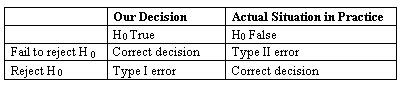#### Subject 4. Type I and Type II Errors in Hypothesis Testing

Because hypothesis tests are heavily dependent on the samples used as "evidence," it is definitely possible, in the case of a bad sample, to make an error in the conclusion of a test.

When a hypothesis is tested, there are four possible outcomes:

• Reject the null hypothesis when it's false. This is a correct decision.
• Incorrectly reject the null hypothesis when it's correct. This is known as a Type I error. The probability of a Type I error is designated by the Greek letter alpha (α) and is called the Type I error rate.
• Don't reject the null hypothesis when it's true. This is a correct decision.
• Don't reject the null hypothesis when it's false. This is known as a Type II error. The probability of a Type II error (the Type II error rate) is designated by the Greek letter beta (β).A Type II error is only an error in the sense that an opportunity to reject the null hypothesis correctly was lost. It is not an error in the sense that an incorrect conclusion was drawn, since no conclusion is drawn when the null hypothesis is not rejected. It has nothing to do with α, other than the fact that it moves in the opposite direction from α (that is, the bigger the one, the smaller the other).

A Type I error, on the other hand, is an error in every sense of the word. A conclusion is drawn that the null hypothesis is false when, in fact, it is true. Therefore, Type I errors are generally considered more serious than Type II errors. The probability of a Type I error (α) is called the significance level; it is set by the experimenter. For example, a 5% level of significance means that there is a 5% probability of rejecting the null hypothesis when it is true.

There is a tradeoff between Type I and Type II errors. The more an experimenter protects him or herself against Type I errors by choosing a low level, the greater the chance of a Type II error. Requiring very strong evidence to reject the null hypothesis makes it very unlikely that a true null hypothesis will be rejected. However, it increases the chance that a false null hypothesis will not be rejected, thus lowering its power. The Type I error rate is almost always set at 0.05 or at 0.01, the latter being more conservative (since it requires stronger evidence to reject the null hypothesis at the 0.01 level then at the 0.05 level).

To reduce the probabilities of both types of errors simultaneously, the sample size n must be increased.